Question

# blem 5 (2000): The closed-loop system is given below. Controller El(s) ) (5% o) Find the system transfer function and discuss the range of Ko to make the stem stable assuming Kp-5. ) (5 %) Find t...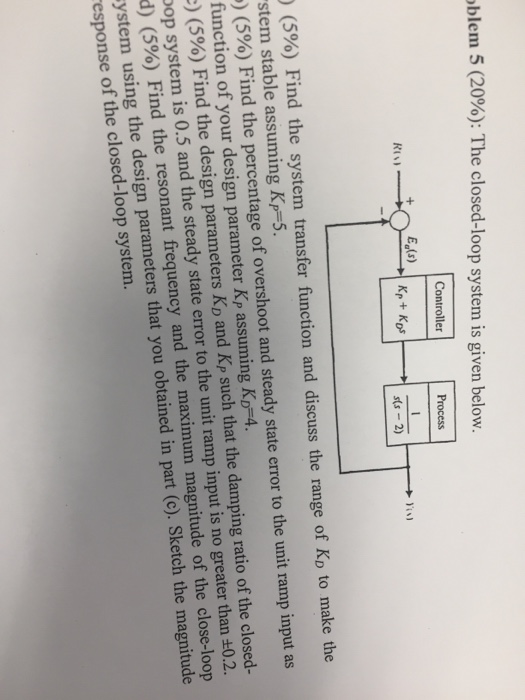blem 5 (2000): The closed-loop system is given below. Controller El(s) ) (5% o) Find the system transfer function and discuss the range of Ko to make the stem stable assuming Kp-5. ) (5 %) Find the percentage of overshoot and steady state error to the unit ramp input as function of your design parameter Kp assuming KD-4. :) (5%) Find the design parameters KD and Kp such that the damping ratio of the closed- pop system is 0.5 and the steady state error to the unit ramp input is no greater than +0.2. d) (5%) Find the resonant frequency and the maximum magnitude of the close-loop ystem using the design parameters that you obtained in part (c). Sketch the magnitude esponse of the closed-loop system.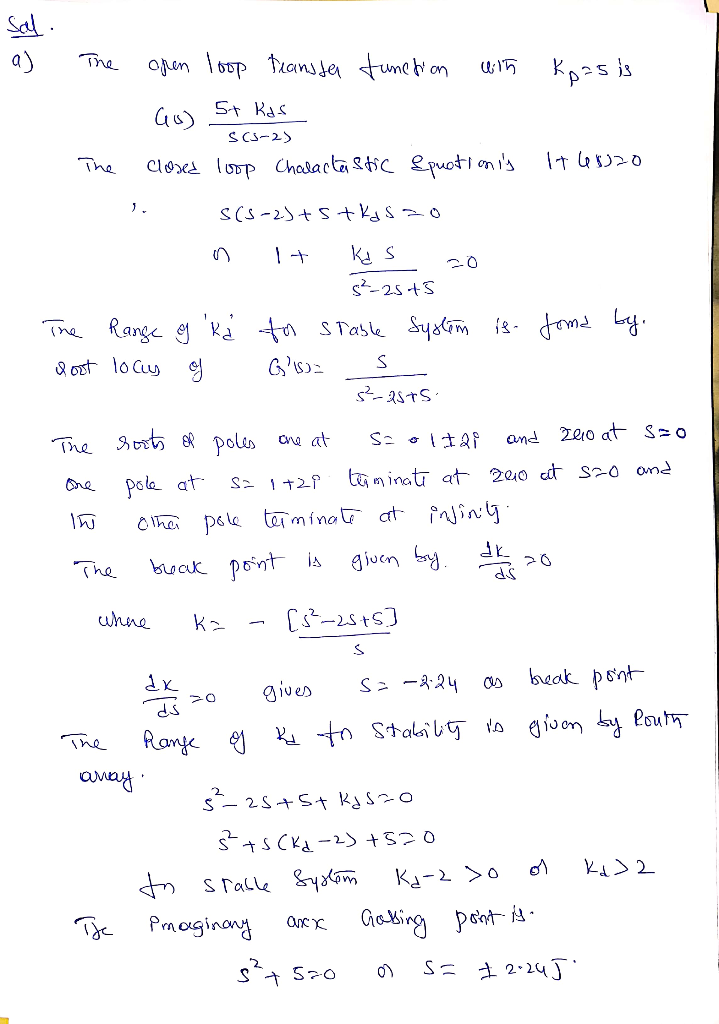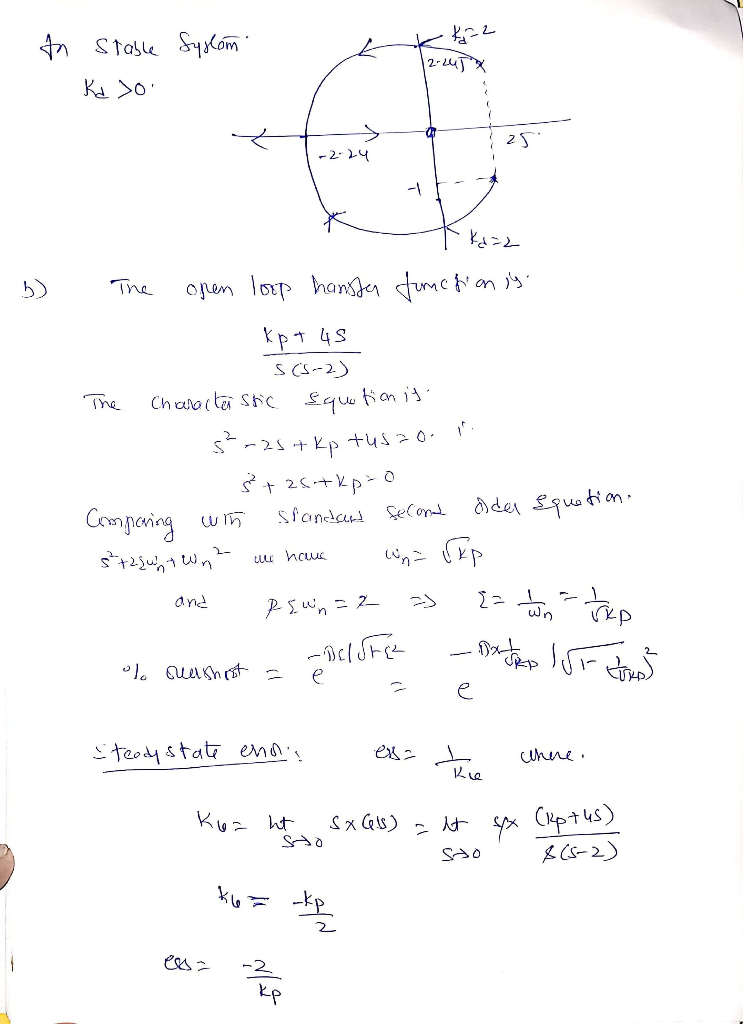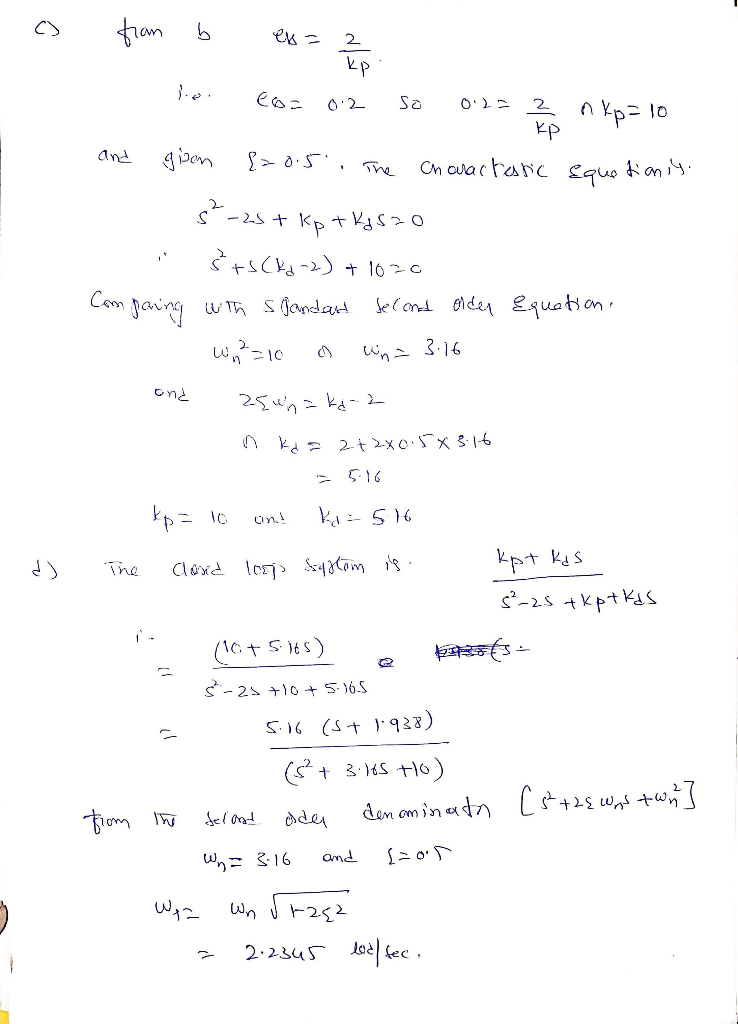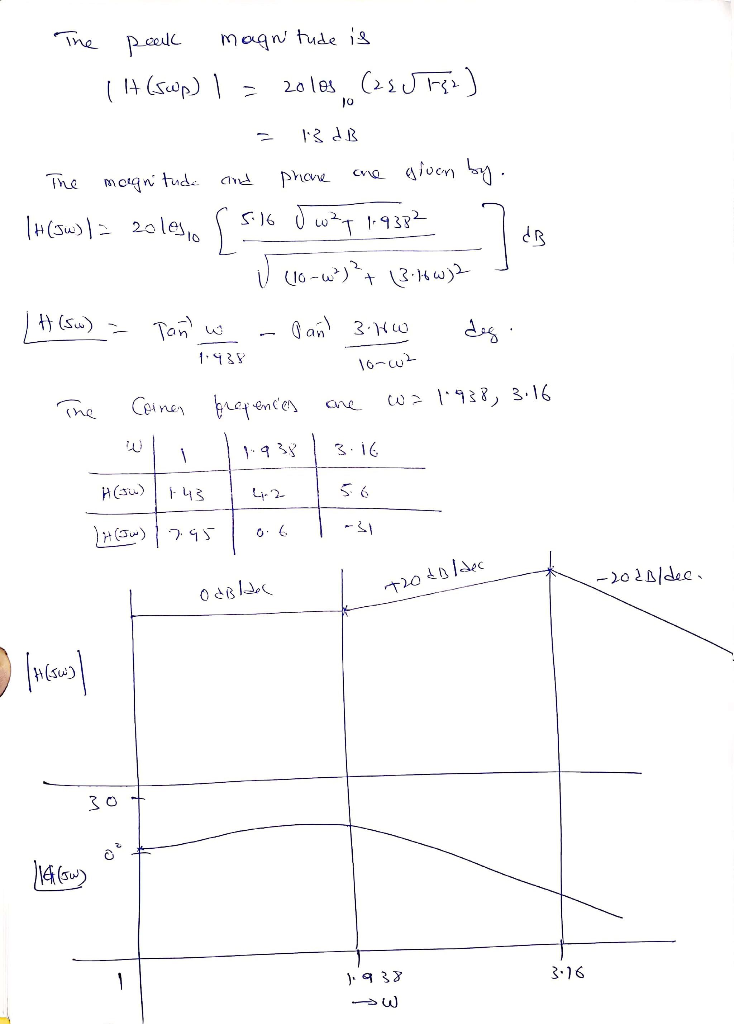#### Earn Coins

Coins can be redeemed for fabulous gifts.

Similar Homework Help Questions
• ### muibliam 5(20%). The closed-loop system is given below. Controller (a) (S%) Find the system transfer function and discuss the range of Ko to make the eystom stuibie assuming K (t)(S%) Find the...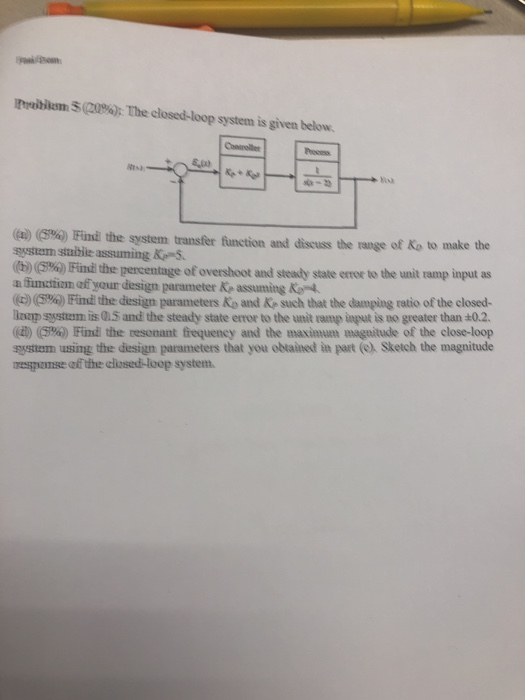muibliam 5(20%). The closed-loop system is given below. Controller (a) (S%) Find the system transfer function and discuss the range of Ko to make the eystom stuibie assuming K (t)(S%) Find the percentage of overshoot and stendy state error to the unit ramp input as a function of your design parameter Ke assuming K4 ( d) 5%) Find hed sagn parameters Ko and Kr such that the damping ratio of the closed- lonp system is O15 and the steady state...

• ### Consider the closed loop system defined by the following block diagram. a) Compute the transfer function...Consider the closed loop system defined by the following block diagram. a) Compute the transfer function E(s)/R(s). b) Determine the steady state error for a unit-step 1. Controller ant Itly Ro- +- HI- 4단Toy , c) d) e) reference input signal. Determine the steady state error response for a unit-ramp reference input signal. Determine the locations of the closed loop poles of the system. Select system parameters kp and ki in terms of k so that damping coefficient V2/2 and...

• ### yUCni ias the block diagram shown below. Controller Process Sensor (a) (5%) Sketch the root locus of the closed-loop system. (b) (5%) Determine the range of K that the closed-loop system is stabl...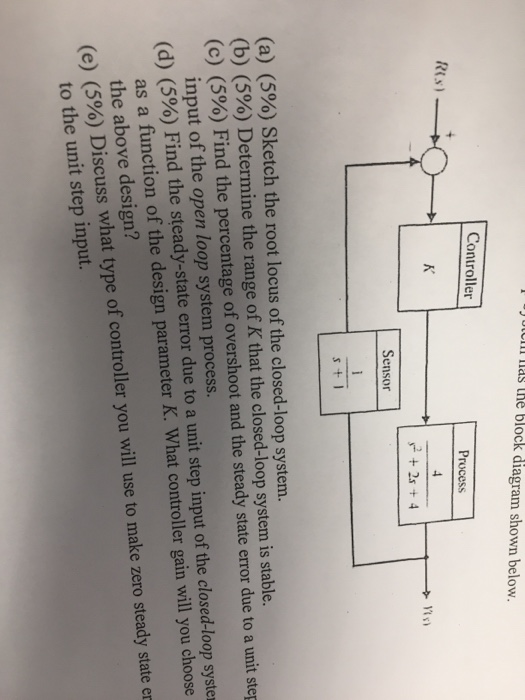yUCni ias the block diagram shown below. Controller Process Sensor (a) (5%) Sketch the root locus of the closed-loop system. (b) (5%) Determine the range of K that the closed-loop system is stable. (c) (5%) Find the percentage of overshoot and the steady state error due to a unit step input of the open loop system process. (d) (5%) Find the steady-state error due to a unit step input of the closed-loop syste as a function of the design parameter...

• ### PROBLEMA: (25%) A closed-loop control system is shown below Ds) T(O) U(A) C(s) (a) Show that a proportional controller (C(s)-kp) will never make the closed-loop system stable. (8%) (Hint: you nee...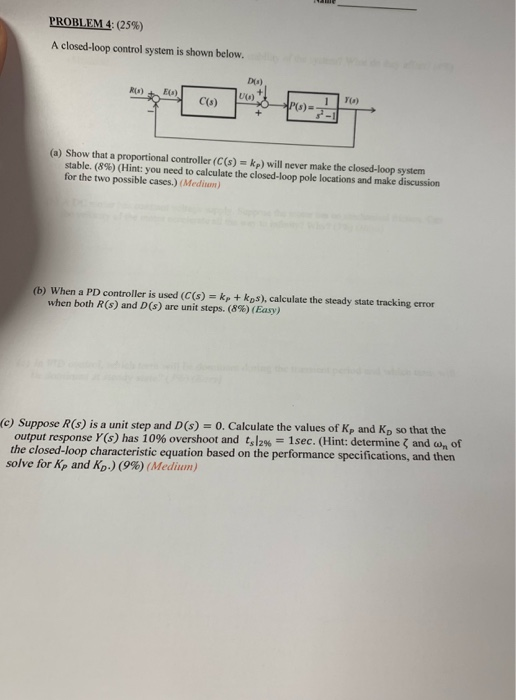PROBLEMA: (25%) A closed-loop control system is shown below Ds) T(O) U(A) C(s) (a) Show that a proportional controller (C(s)-kp) will never make the closed-loop system stable. (8%) (Hint: you need to calculate the closed-loop pole locations and make discussion for the two possible cases.) (Medim) (b) When a PD controller is used (C(s)kp+ kps), calculate the steady state tracking error when both R(s) and D(s) are unit steps. (8%) (Easy) (e) Suppose R(s) is a unit step and D(s)...

• ### Problem 3 (25%): The closed-loop system has the block diagram shown below. Controlle Process Sensor s + l (a) (5%) Sketch the root locus of the closed-loop system. (b) (5%) Determine the range of...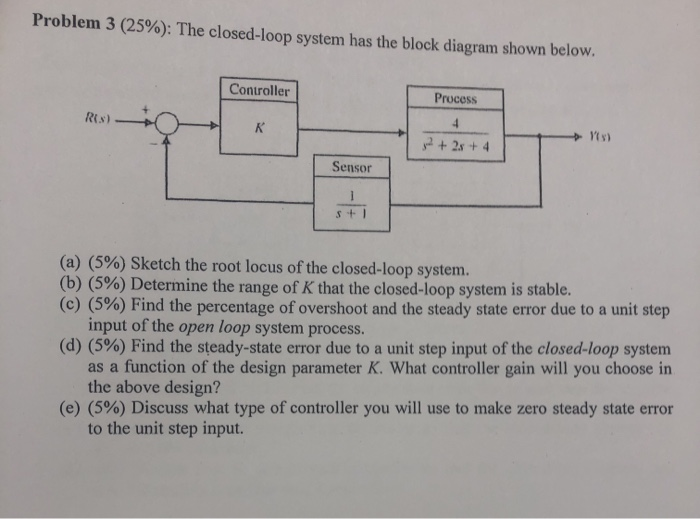Problem 3 (25%): The closed-loop system has the block diagram shown below. Controlle Process Sensor s + l (a) (5%) Sketch the root locus of the closed-loop system. (b) (5%) Determine the range of K that the closed-loop system is stable. (c) (5%) Find the percentage of overshoot and the steady state error due to a unit step input of the open loop system process. (d) (5%) Find the steady-state error due to a unit step input of the closed-loop...

• ### A unity feedback system with the forward transfer function G)2)(s +5) is operating with a closed-...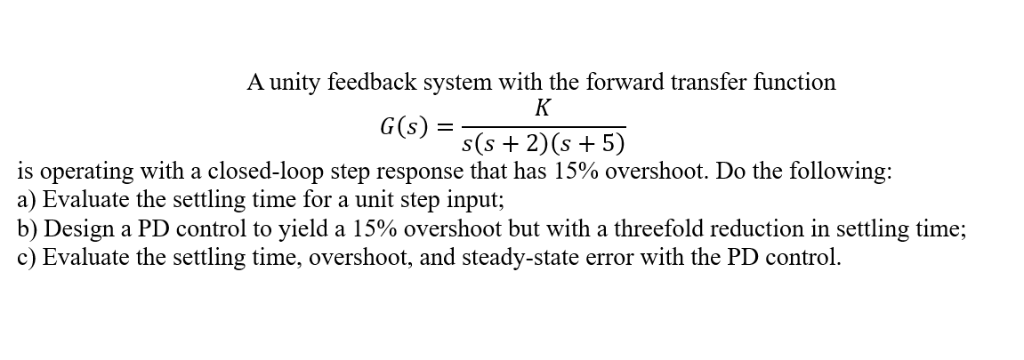A unity feedback system with the forward transfer function G)2)(s +5) is operating with a closed-loop step response that has 15% overshoot. Do the following: a) Evaluate the settling time for a unit step input; b) Design a PD control to yield a 15% overshoot but with a threefold reduction in settling time; c) Evaluate the settling time, overshoot, and steady-state error with the PD control. A unity feedback system with the forward transfer function G)2)(s +5) is operating with...

• ### PROBLEM: A unity feedback system with the forward transfer function K G(s) s(s+7) is operating with...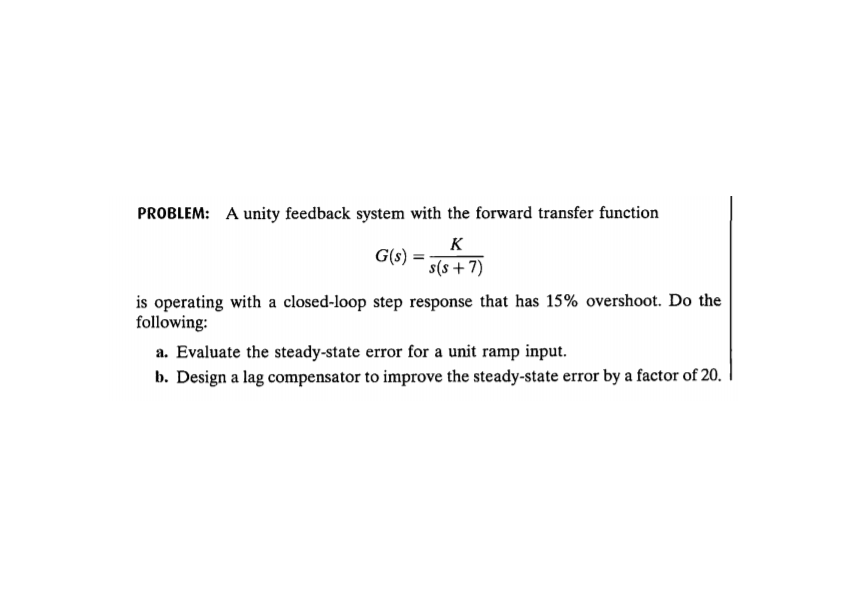PROBLEM: A unity feedback system with the forward transfer function K G(s) s(s+7) is operating with a closed-loop step response that has 15% overshoot. Do the following: a. Evaluate the steady-state error for a unit ramp input. b. Design a lag compensator to improve the steady-state error by a factor of 20. c. Evaluate the steady-state error for a unit ramp input to your compensated system. d. Evaluate how much improvement in steady-state error was realized.

• ### A unity feedback system with the forward transfer function G(s)=K/(s+1)(s+3)(s+6) is operating wi...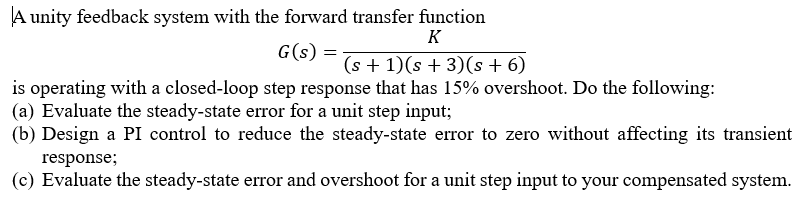A unity feedback system with the forward transfer function G(s)=K/(s+1)(s+3)(s+6) is operating with a closed-loop step response that has 15% overshoot. Do the following: a) Evaluate the steady-state error for a unit step input b) Design a PI control to reduce the steady-state error to zero without affecting its transient response c) Evaluate the steady-state error and overshoot for a unit step input to your compensated system A unity feedback system with the forward transfer function G(s) is operating with...

• ### Given the system shown below find the closed loop transfer function, then find the system type...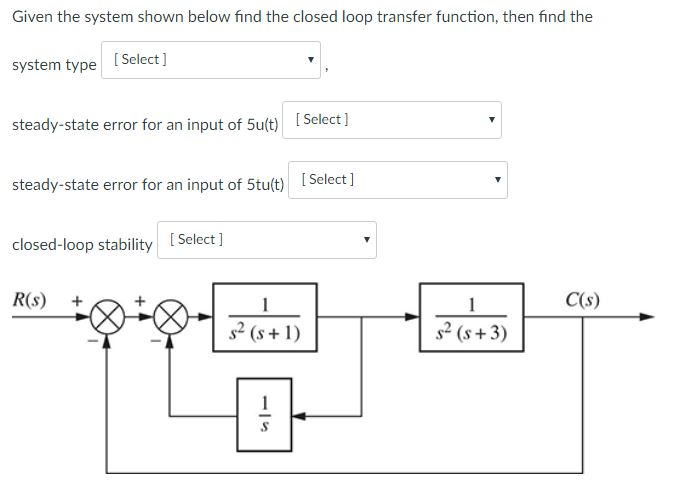Given the system shown below find the closed loop transfer function, then find the system type Selectj steady-state error for an input of 5ut)Select] steady-state error for an input of5tt[Select 1 closed-loop stablity Select ] R(s) [Select ] 1 C(s) s2 (s+1) s2 (s +3)

• ### For the closed-loop system shown, and given: Design a PD Controller i.e. where  C(s)=Kp+Kds to satisfy the following specifications: For the closed-loop system shown, and given: 26.40 821.25 s+12.00...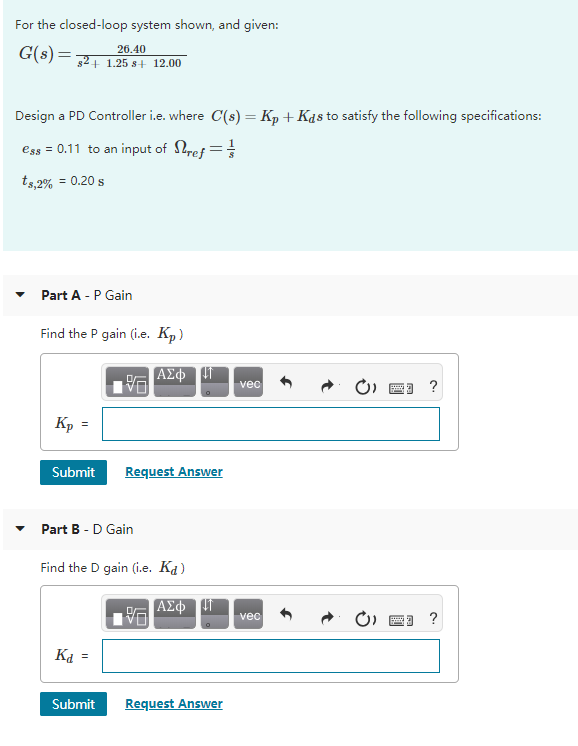For the closed-loop system shown, and given: Design a PD Controller i.e. where  C(s)=Kp+Kds to satisfy the following specifications: For the closed-loop system shown, and given: 26.40 821.25 s+12.00 Design a PD Controller i.e. where C(s) - Kp+ Kas to satisfy the following specifications: ess 0.11 to an input of Stref 4.2% :0.20 s Part A P Gain Find the P gain ie. Kp) vec Submit Request Answer Part B-D Gain Find the D gain Gie. Kd) vec Kd Submit...